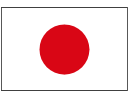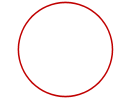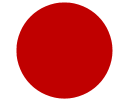Search IntMath
Close

450+ Math Lessons written by Math Professors and Teachers

5 Million+ Students Helped Each Year

1200+ Articles Written by Math Educators and Enthusiasts

Simplifying and Teaching Math for Over 23 Years

# What is a circle?

By Murray Bourne, 11 Apr 2011Japanese flag

Most people would describe the Japanese flag as being "a red circle on a white background". But is it really, mathematically speaking?

Reader Irshad Hussain recently asked for "a clear definition of a circle." He wondered if the circle is only a boundry or does it include the whole interior also?

When you think "circle", do you see a curve, like this:Or do you think of it as a region, like this?Math Open Reference defines a circle as:

A line forming a closed loop, every point on which is a fixed distance from a center point.

This is the first diagram above.

The American Heritage Science Dictionary gives the following definition, also considering the circle as a curve, not a region:

A closed curve whose points are all on the same plane and at the same distance from a fixed point (the center).

Wolfram|Alpha also defines it as a plane curve. (And that's all. Even though it lists several important equations for circles, no mention is made of the property of equidistance from a point).

Google's definitions cover both cases, but give precedence to the region definition (the second diagram):

1. A round plane figure whose boundary (the circumference) consists of points equidistant from a fixed point (the center)
2. The line enclosing such a figure

Here's a definition that gives a broader view:

Ellipse in which the two axes are of equal length.

One of the silliest definitions is from the The American Heritage Dictionary:

Circle: A planar region bounded by a circle.

How can an object be bounded by itself? One could argue the definition itself is circular. 🙂

## Is the circular region a disk?

The simplest solution is to define a circle as a plane curve and a disk as a plane region, bounded by a circle. However, "disk" to me suggests a 3-dimensional object (a very flat cylinder).

What are your thoughts on how we should define a cirlce?

### 7 Comments on “What is a circle?”

1. Dalcde says:

I believe that a circle is a curve, mathematically. We talk about "equation of a circle" which defines a curve (the region enclosed would be described by x^2+y^2<r^2). The region inside the curve is a disk (or disc).

In our everyday life, however, a circle can refer to both the curve and the region enclosed in the curve.

In everyday use, the term "circle" may be used interchangeably to refer to either the boundary of the figure, or to the whole figure including its interior; in strict technical usage, the circle is the former and the latter is called a disk.

2. Yan Kow Cheong says:

Based on the "abnormally high" number of theorems on the circle, no doubt the circle is often taken to be the most beautiful curve in the all of mathematics - simple but not simplistic.

There are enough properties of (and thousands of conjectures to work on) the circle to keep one mathematically busy for a three- or four-scores-and-ten lifespan.

Let me end on a spiritual note:

"The nature of God is a circle of which the center is everywhere and the circumference is nowhere." Anonymous

Circularly yours

3. Mehdi says:

The easiest way to define a circle is "The locus of points equidistant from a fixed point i.e. the center of the circle"...
Also x^2+y^2=r^2 implies that only the boundary and not the region inside the circle

4. MJ56003 says:

But didn't we all have classes that asked "What is the area of this circle?" and "What is the circumference of this circle?"

If a circle is only a line, then it would have an area of zero.

5. j says:

A circle is the locus of a point on which all points are equidistant from a fixed internal point known as the center.(sorry, for grammatical mistakes, if any)

6. Eric Meacham says:

Circle,1.any object which is perfectly round; ie. ⊙
2. Used in mathematics,social sciences, religion, astronomy, literature, and various forms of sports and arts , often deviating from the first definition .
3. Beginning at any point , going in 360° .

7. Bill says:

Three ways to define a circle mathematically. 1)Locus of points:The set of points in a plane equidistant from a fixed point called the center.
2)Conic Sections:The intersection of a plane and a right circular cone such that the plane is perpendicular to the axis of the cone.
3)Algebraically:The set of ordered pairs satisfying the equation (x-h)^2+(y-k)^2=r^2 where (h,k) is the center and r is the radius.

### Comment Preview

HTML: You can use simple tags like <b>, <a href="...">, etc.

To enter math, you can can either:

1. Use simple calculator-like input in the following format (surround your math in backticks, or qq on tablet or phone):
a^2 = sqrt(b^2 + c^2)
(See more on ASCIIMath syntax); or
2. Use simple LaTeX in the following format. Surround your math with $$ and $$.
$$\int g dx = \sqrt{\frac{a}{b}}$$
(This is standard simple LaTeX.)

NOTE: You can mix both types of math entry in your comment.